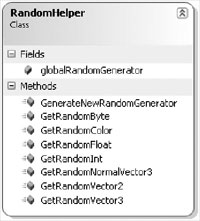# §3.4随机函数（RandomHelper）辅助类

Random randomGenerator = new Random((int)DateTime.Now.Ticks);

Vector3 randomNormalVector = new Vector3( (float)randomGenerator.NextDouble() * 2.0f - 1.0f, (float)randomGenerator.NextDouble() * 2.0f - 1.0f,(float)randomGenerator.NextDouble() * 2.0f - 1.0f);

randomNormalVector.Normalize(); 取代再三重复这段代码，诸如RandomHelper的助手类会非常有用。图3-8显示了RandomHelper类的基本结构。## 生成随机向量

/// <summary>

/// Get Random Vector2 获得二维随机向量

///</summary>

/// <param name="min" /> Minimum for each component </param>

/// <param name="max" /> Maximum for each component</param>

/// <returns>Vector2</returns>

public static Vector2 GetRandomVector2(float min, float max)

{

return new Vector2(GetRandomFloat(min, max),GetRandomFloat(min, max));

} // GetRandomVector2(min, max)

2006 - 2023，推荐分辨率1024*768以上，推荐浏览器Chrome、Edge等现代浏览器，截止2021年12月5日的访问次数：1872万9823 站长邮箱